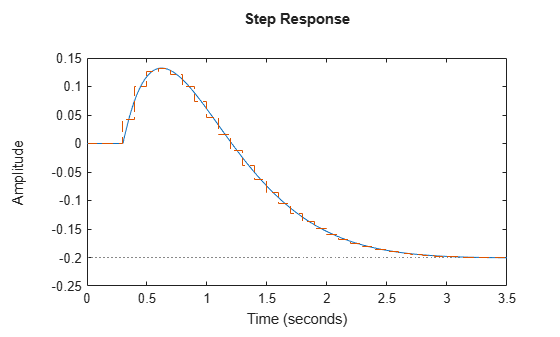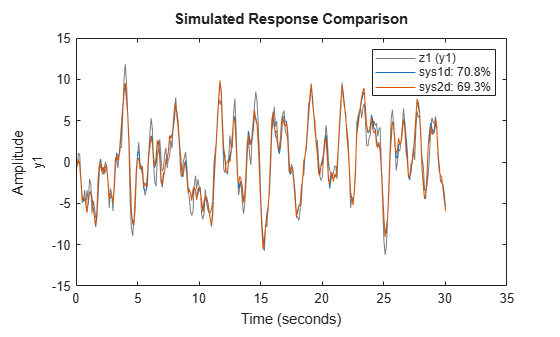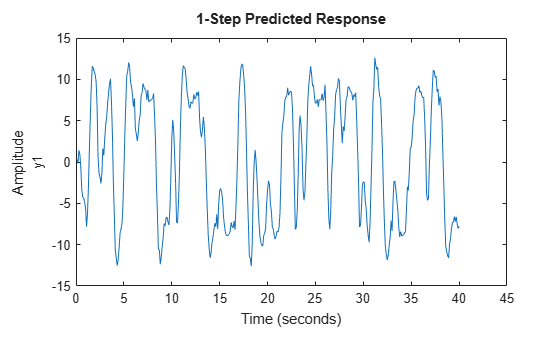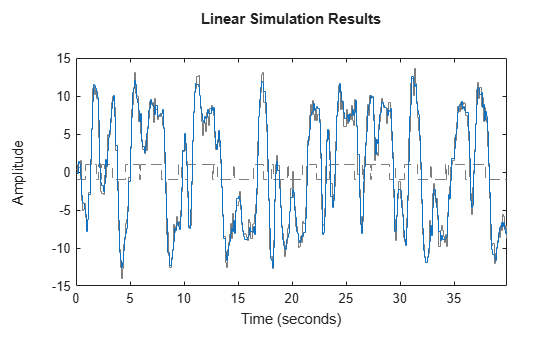# c2d

Convert model from continuous to discrete time

## Syntax

``sysd = c2d(sysc,Ts)``
``sysd = c2d(sysc,Ts,method)``
``sysd = c2d(sysc,Ts,opts)``
``````[sysd,G] = c2d(___)``````

## Description

example

````sysd = c2d(sysc,Ts)` discretizes the continuous-time dynamic system model `sysc` using zero-order hold on the inputs and a sample time of `Ts`.```

example

````sysd = c2d(sysc,Ts,method)` specifies the discretization method.```

example

````sysd = c2d(sysc,Ts,opts)` specifies additional options for the discretization.```
``````[sysd,G] = c2d(___)```, where `sysc` is a state-space model, returns a matrix, `G` that maps the continuous initial conditions x0 and u0 of the state-space model to the discrete-time initial state vector x.```

## Examples

collapse all

Discretize the following continuous-time transfer function:

`$H\left(s\right)={e}^{-0.3s}\frac{s-1}{{s}^{2}+4s+5}.$`

This system has an input delay of 0.3 s. Discretize the system using the triangle (first-order-hold) approximation with sample time `Ts` = 0.1 s.

```H = tf([1 -1],[1 4 5],'InputDelay', 0.3); Hd = c2d(H,0.1,'foh');```

Compare the step responses of the continuous-time and discretized systems.

`step(H,'-',Hd,'--')`Discretize the following delayed transfer function using zero-order hold on the input, and a 10-Hz sampling rate.

`$H\left(s\right)={e}^{-0.25s}\frac{10}{{s}^{2}+3s+10}.$`

```h = tf(10,[1 3 10],'IODelay',0.25); hd = c2d(h,0.1)```
```hd = 0.01187 z^2 + 0.06408 z + 0.009721 z^(-3) * ---------------------------------- z^2 - 1.655 z + 0.7408 Sample time: 0.1 seconds Discrete-time transfer function. ```

In this example, the discretized model `hd` has a delay of three sampling periods. The discretization algorithm absorbs the residual half-period delay into the coefficients of `hd`.

Compare the step responses of the continuous-time and discretized models.

`step(h,'--',hd,'-')`Create a continuous-time state-space model with two states and an input delay.

```sys = ss(tf([1,2],[1,4,2])); sys.InputDelay = 2.7```
```sys = A = x1 x2 x1 -4 -2 x2 1 0 B = u1 x1 2 x2 0 C = x1 x2 y1 0.5 1 D = u1 y1 0 Input delays (seconds): 2.7 Continuous-time state-space model. ```

Discretize the model using the Tustin discretization method and a Thiran filter to model fractional delays. The sample time `Ts` = 1 second.

```opt = c2dOptions('Method','tustin','FractDelayApproxOrder',3); sysd1 = c2d(sys,1,opt)```
```sysd1 = A = x1 x2 x3 x4 x5 x1 -0.4286 -0.5714 -0.00265 0.06954 2.286 x2 0.2857 0.7143 -0.001325 0.03477 1.143 x3 0 0 -0.2432 0.1449 -0.1153 x4 0 0 0.25 0 0 x5 0 0 0 0.125 0 B = u1 x1 0.002058 x2 0.001029 x3 8 x4 0 x5 0 C = x1 x2 x3 x4 x5 y1 0.2857 0.7143 -0.001325 0.03477 1.143 D = u1 y1 0.001029 Sample time: 1 seconds Discrete-time state-space model. ```

The discretized model now contains three additional states `x3`, `x4`, and `x5` corresponding to a third-order Thiran filter. Since the time delay divided by the sample time is 2.7, the third-order Thiran filter (`'FractDelayApproxOrder'` = 3) can approximate the entire time delay.

Estimate a continuous-time transfer function, and discretize it.

```load iddata1 sys1c = tfest(z1,2); sys1d = c2d(sys1c,0.1,'zoh');```

Estimate a second order discrete-time transfer function.

`sys2d = tfest(z1,2,'Ts',0.1);`

Compare the response of the discretized continuous-time transfer function model, `sys1d`, and the directly estimated discrete-time model, `sys2d`.

`compare(z1,sys1d,sys2d)`The two systems are almost identical.

Discretize an identified state-space model to build a one-step ahead predictor of its response.

Create a continuous-time identified state-space model using estimation data.

```load iddata2 sysc = ssest(z2,4);```

Predict the 1-step ahead predicted response of `sysc`.

`predict(sysc,z2)`Discretize the model.

`sysd = c2d(sysc,0.1,'zoh');`

Build a predictor model from the discretized model, `sysd`.

```[A,B,C,D,K] = idssdata(sysd); Predictor = ss(A-K*C,[K B-K*D],C,[0 D],0.1);```

`Predictor` is a two-input model which uses the measured output and input signals `([z1.y z1.u])` to compute the 1-step predicted response of `sysc`.

Simulate the predictor model to get the same response as the `predict` command.

`lsim(Predictor,[z2.y,z2.u])`The simulation of the predictor model gives the same response as `predict(sysc,z2)`.

## Input Arguments

collapse all

Continuous-time model, specified as a dynamic system model such as `idtf`, `idss`, or `idpoly`. `sysc` cannot be a frequency response data model. `sysc` can be a SISO or MIMO system, except that the `'matched'` discretization method supports SISO systems only.

`sysc` can have input/output or internal time delays; however, the `'matched'`, `'impulse'`, and `'least-squares'` methods do not support state-space models with internal time delays.

The following identified linear systems cannot be discretized directly:

• `idgrey` models whose `FunctionType` is `'c'`. Convert to `idss` model first.

• `idproc` models. Convert to `idtf` or `idpoly` model first.

Sample time, specified as a positive scalar that represents the sampling period of the resulting discrete-time system. `Ts` is in `TimeUnit`, which is the `sysc.TimeUnit` property.

Discretization method, specified as one of the following values:

• `'zoh'` — Zero-order hold (default). Assumes the control inputs are piecewise constant over the sample time `Ts`.

• `'foh'` — Triangle approximation (modified first-order hold). Assumes the control inputs are piecewise linear over the sample time `Ts`.

• `'impulse'` — Impulse invariant discretization

• `'tustin'` — Bilinear (Tustin) method. To specify this method with frequency prewarping (formerly known as the `'prewarp'` method), use the `PrewarpFrequency` option of `c2dOptions`.

• `'matched'` — Zero-pole matching method

• `'least-squares'` — Least-squares method

• `'damped'` — Damped Tustin approximation based on the `TRBDF2` formula for sparse models only.

For information about the algorithms for each conversion method, see Continuous-Discrete Conversion Methods.

Discretization options, specified as a `c2dOptions` object. For example, specify the prewarp frequency, order of the Thiran filter or discretization method as an option.

## Output Arguments

collapse all

Discrete-time model, returned as a dynamic system model of the same type as the input system `sysc`.

When `sysc` is an identified (IDLTI) model, `sysd`:

• Includes both measured and noise components of `sysc`. The innovations variance λ of the continuous-time identified model `sysc`, stored in its `NoiseVariance`property, is interpreted as the intensity of the spectral density of the noise spectrum. The noise variance in `sysd` is thus λ/Ts.

• Does not include the estimated parameter covariance of `sysc`. If you want to translate the covariance while discretizing the model, use `translatecov`.

Mapping of continuous-time initial conditions x0 and u0 of the state-space model `sysc` to the discrete-time initial state vector x, returned as a matrix. The mapping of initial conditions to the initial state vector is as follows:

`$x\left[\text{ }0\right]=G\cdot \left[\begin{array}{c}{x}_{0}\\ {u}_{0}\end{array}\right]$`

For state-space models with time delays, `c2d` pads the matrix `G` with zeroes to account for additional states introduced by discretizing those delays. See Continuous-Discrete Conversion Methods for a discussion of modeling time delays in discretized systems.

## Version History

Introduced before R2006a